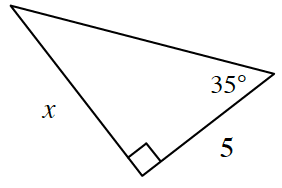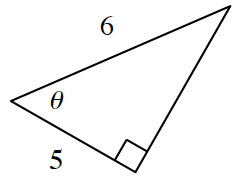### Home > INT3 > Chapter 10 > Lesson 10.3.2 > Problem10-166

10-166.

This problem is a checkpoint for solving triangles. It will be referred to as Checkpoint 10.

1. Solve for $x$.1. Solve for $θ$.1. Calculate the area of $∆ABC$ if $AB = 17, AC = 12$, and $m∠CAB = 48^\circ$.

2. Rajeet is creating plans for a triangular rock garden in his backyard. Two sides of the garden are $5.0$ feet and $7.0$ feet. If the angle between those sides is $80^\circ$, how long is the third side?

Check your answers by referring to the Checkpoint 10 materials located at the back of your book.

Ideally at this point you are comfortable working with these types of problems and can solve them correctly. If you feel that you need more confidence when solving these types of problems, then review the Checkpoint 10 materials and try the practice problems provided. From this point on, you will be expected to do problems like these correctly and with confidence.

Answers and extra practice are located in the back of your printed textbook or in the Reference Tab of your eBook. If you have an eBook for Int3, login and then click the following link: Checkpoint 10: Solving Triangles